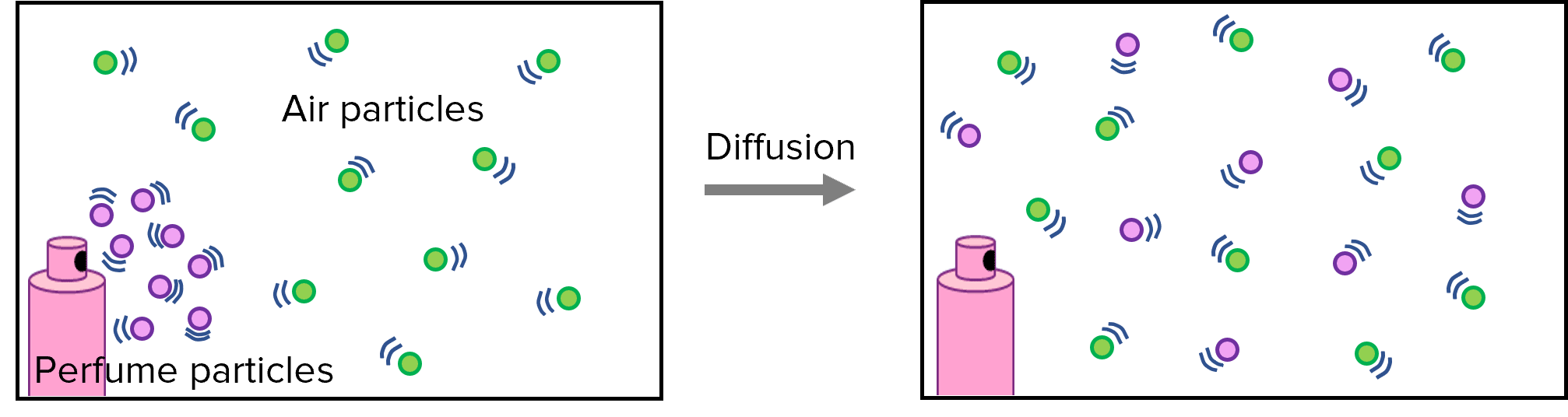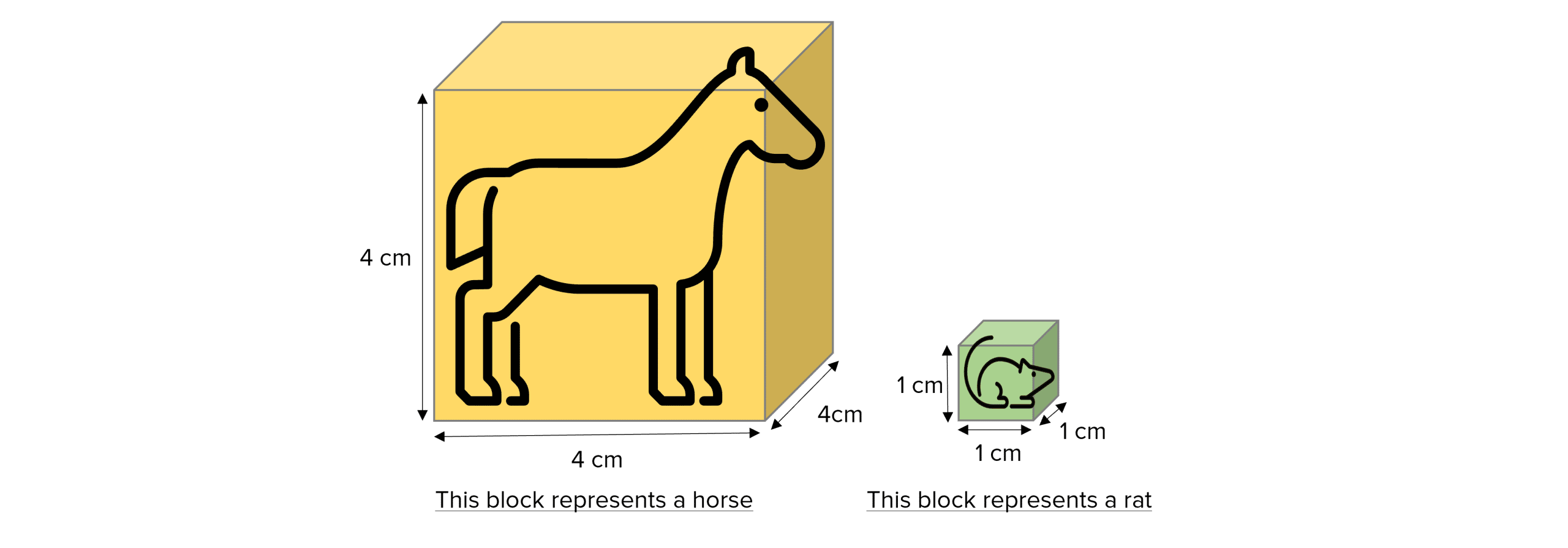# Diffusion

GCSEAQABiology FoundationBiology HigherCombined Science FoundationCombined Science Higher

## Diffusion

Diffusion describes the spreading out of particles and is especially important to living cells. Cell membranes allow for the diffusion of substances in and out of cells which keeps them alive. The rate of diffusion across a membrane can be affected by many different factors.

## What is Diffusion?

Particles move around randomly and so spread out evenly in a certain space. They do this by moving from an area where there is lots of them (higher concentration) to an area where there is few of them (lower concentration), down a concentration gradient.

A good example of this is spraying a perfume bottle in a room:Diffusion is the term used to describe this movement and you will need to remember its definition:

Diffusion is the net movement of particles from an area of higher concentration to an area of lower concentration.

Diffusion happens in both solutions and gases as the particles are free to move randomly.

Diffusion of water is called osmosis.

GCSECombined Science FoundationCombined Science HigherBiology FoundationBiology HigherAQA

## Diffusion Across a Cell Membrane

Substances move in and out of cells by diffusing across the cell membrane from areas of high concentrations to low concentrations.

Cell membranes are partially permeable which means only certain substances are able to diffuse across them and move in and out of the cell.

Some common examples include:

• Oxygen and carbon dioxide diffuse in and out of cells during gas exchange.
• Waste products such as urea diffuse out of cells and into the blood plasma so it can be excreted in the kidney.
• Some proteins and big carbohydrates from food are too large to diffuse across a membrane and into the bloodstream so they get digested into amino acids and glucose which are small enough to diffuse.GCSECombined Science FoundationCombined Science HigherBiology FoundationBiology HigherAQA

## Rate of Diffusion

The rate of diffusion is simply how much substance is diffused in a given time.

The rate of diffusion is affected by:

• Temperature – rate of diffusion is faster at higher temperatures because the particles will have more kinetic energy, thus will move around faster.
• Concentration gradient – the bigger the difference between the concentrations of each side, the faster the rate of diffusion.
• Surface area of the membrane – the larger the surface area, the faster the rate of diffusion because more particles can pass through the membrane at the same time.
GCSECombined Science FoundationCombined Science HigherBiology FoundationBiology HigherAQA

## Surface Area to Volume Ratio

Surface area to volume ratio (SA:V) is used to measure how easily substances can move in and out of an organism.

Single-celled organisms like bacteria have a very large surface area to volume ratios because their outer surface area is large in comparison to its volume. This means substances do not have to travel far to get where they need to be within the cell and simple methods of diffusion, osmosis and active transport are sufficient to keep the cell alive.

Multi-cellular organisms, like plants and mammals, have very small surface area to volume ratios because their surface area is small in comparison to their volume and the distance between the surface and centre of the organism is large. This means they require highly adapted exchange surfaces and transport systems to provide the cells with everything they require.

GCSECombined Science FoundationCombined Science HigherBiology FoundationBiology HigherAQA

## Calculating and Comparing Surface Area to Volume Ratio

We can calculate surface area to volume ratio by modelling different organisms with blocks.

To do this you need to calculate the total surface area and the volume of the block.

• Total surface area is achieved by calculating the surface area of each side of the shape $\text{(Length} \times \text{Width)}$ and adding them all together.
• Volume is calculated by doing $\text{Length} \times\text{Width}\times\text{Height}$ of the block.

The surface area to volume ratio of different organisms can only be compared to each other when the initial measurements are taken with the same units.

Example: Calculate the surface area to volume ratios for the horse and the rat depicted below.Horse:

$\text{Total Surface Area} =\textcolor{ffad05}{4}^2 \times 6= 96\text{ cm}^2$

$\text{Volume}= \textcolor{ffad05}{4}\times \textcolor{ffad05}{4}\times\textcolor{ffad05}{4} = 64\text{ cm}^3$

$\text{SA:V} = 96:64 = \textcolor{ffad05}{1.5:1}$

Rat

$\text{Total Surface Area} =\textcolor{7cb447}{1}^2 \times 6= 6\text{ cm}^2$

$\text{Volume}= \textcolor{7cb447}{1}\times \textcolor{7cb447}{1}\times\textcolor{7cb447}{1} = 1\text{ cm}^3$

$\text{SA:V} = \textcolor{7cb447}{6:1}$

The SA:V of the small rat is much greater than the SA:V of the large horse.

GCSECombined Science FoundationCombined Science HigherBiology FoundationBiology HigherAQA

## Diffusion Example Questions

Diffusion is the net movement of particles from an area of high concentration to low (down a concentration gradient).Gold Standard Education

1. Temperature – Rate of diffusion increases with increasing temperature because the particles have more kinetic energy so move around faster.
2. Concentration gradient – The bigger the difference in the concentrations between each area, the faster the rate of diffusion.
3. Surface area – the larger the surface area the faster the rate of diffusion because more particles can pass through at once.Gold Standard Education

$\text{Total Surface Area} =2^2 \times 6= 24\text{ μm}^2$

$\text{Volume}= 2\times 2\times2 = 8\text{ μm}^3$

$\text{SA:V} = 24:8 = 3:1$Gold Standard Education

## Diffusion Worksheet and Example Questions

GCSEOfficial MMEProduct